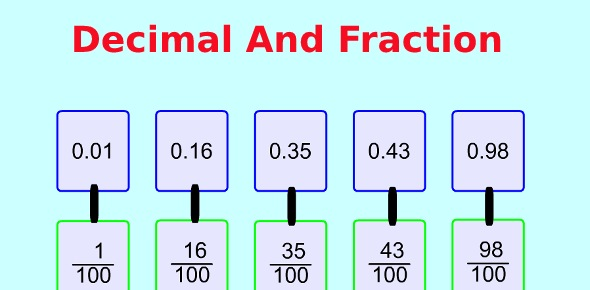# Fractions And Decimals

5 Questions | Total Attempts: 174SettingsThis quiz is to help 4th grade student's develop equivalent relationships between commonly used fractions, decmals, and whole numbers.

Related Topics
• 1.
1/2 is equal to which decimal value?
• A.

0.55

• B.

0.5

• C.

0.6

• D.

0.10

• 2.
4/2 is equal to which whole number value?
• A.

4

• B.

3

• C.

2

• D.

6

• 3.
Which of the following answers is another way to state the value of 2?
• A.

2.0

• B.

1.5

• C.

2/2

• D.

4/6

• 4.
.25 is equal to which of the following fraction values?
• A.

4/8

• B.

1/3

• C.

2/5

• D.

1/4

• 5.
9/3 is equal to which whole number value?
• A.

2

• B.

3

• C.

4

• D.

9